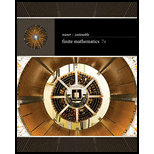# Assume that it is true that “Polly sings well,” it is false that “Quentin writes well,” and it is true that “Rita is good at math.” Determine the truth of each of the statements in Exercises 25–32. Either Polly sings well and Quentin writes poorly, or Rita is good at math.### Finite Mathematics

7th Edition
Stefan Waner + 1 other
Publisher: Cengage Learning
ISBN: 9781337280426### Finite Mathematics

7th Edition
Stefan Waner + 1 other
Publisher: Cengage Learning
ISBN: 9781337280426
Chapter A, Problem 29E
Textbook Problem

## Assume that it is true that “Polly sings well,” it is false that “Quentin writes well,” and it is true that “Rita is good at math.” Determine the truth of each of the statements in Exercises 25–32.Either Polly sings well and Quentin writes poorly, or Rita is good at math.

Expert Solution
To determine

The truth of the statement “Either Polly sings well and Quentin writes poorly, or Rita is good at math.” where it is assumed that the statement “Poly sings well” is true and Quentin writes well is false, and “Rita is good at math” is true.

### Explanation of Solution

A sentence is a statement if it is defined as a declarative sentence and whose value is either true or false which is called its truth value.

If p is a statement, then the negation of the statement is defined as p, called not p.

To represent the new statement from old ones, the conjunction is used such as pq which is p and q.

The truth value of this is true if both p and q are true otherwise, false and Disjunction is used such as pq which is p or q. The truth value of this is true if either p or q is true or both true.

Consider the statement, p: Polly sings well,

The truth value of p is true.

Consider the statement, q: Quentin writes well,

The truth value of q is false.

The statement Quentin writes poorly is represented by the negation of q,

q

The truth value q is true

### Want to see the full answer?

Check out a sample textbook solution.See solution

### Want to see this answer and more?

Bartleby provides explanations to thousands of textbook problems written by our experts, many with advanced degrees!

See solution